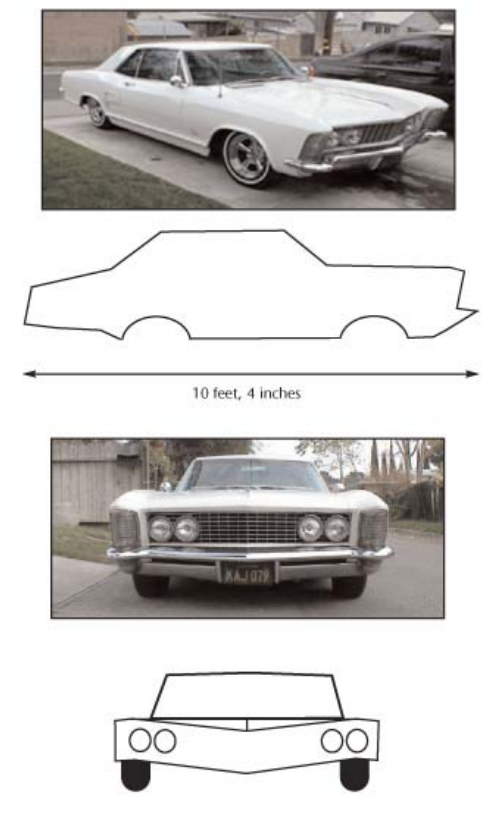Subject:
Mathematics

Topics: Ratios
Common Core State Standard: 6.RP.1, 6.RP.3d, 7.RP.3,
Knowledge and Skills:

• Understands the concept “scale.”
• Can use proportional reasoning to solve problems.

Materials:

Modeling clay

Lesson:

Procedure: This activity should be done by students working in pairs.

Ensure that students understand the task they are being asked to carry out.

To the degree that students can work independently, allow them to do so. If they need more guidance, help them as needed through these steps:

1) Measure the main dimensions on the drawing.

2) Use the indicated dimension to find the scale of the drawing.

3) Multiply all dimensions that you measured in step 1 by the scale factor you found in step 2, in order to get the dimensions of the actual vehicle.

4) Convert the dimensions of the vehicle to inches, then multiply by 1/64 to get the dimensions of the model.

5) Make the model with those dimensions.

### The 1963 Buick Riviera

The image and diagrams below depict the 1963 Buick Riviera. The drawings are to scale.

Use the information provided to make, from clay, an accurate model of the car at 1/64 scale.